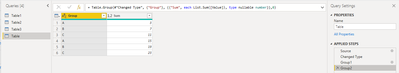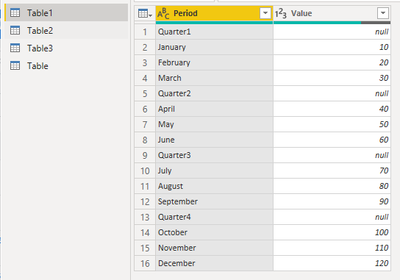cancel
Showing results for
Did you mean:## Partial Grouping in Power Query

As for global grouping, you should be familiar with it as follows:This article explains how to use power query for partial grouping. The partial mode is to search in the condition column from top to bottom, and group consecutive and identical items according to different conditions, as shown below:Partial grouping involves the fourth parameter of Table.Group . Since the default is global mode, the fourth parameter can be omitted, and the effect is the same. To enable partial mode, you need to specify 0 for the fourth parameter, the fifth parameter as the conditional judgment grouping, and the second parameter as a custom function.

Below I will use three scenarios to explain the use of partial grouping:

Scenario 1:

Table1:Based on the above table, our requirement is to group by each ‘Quarter’ and get the sum of corresponding values. We need to search in the ‘Period’ column from top to bottom to find the corresponding quarter and months for that quarter. Here is the formula:

``= Table.Group(#"Changed Type","Period",{"Sum",each List.Sum(_[Value])},0,(x,y)=>Number.From(Text.StartsWith(y,"Quarter")))``

Since we need to use partial group, the fourth parameter needs to be set as 0. The fifth parameter is the most important. It is a function that represents the logic of group. ‘x’ represents the current item and ‘y’ represents all the items below compared to the current item. From the first item, it will find all period values until the period values satisfy the condition ‘Text.StartsWith(y,"Quarter")’.

Result:Scenario 2:

Table2:Based on the above table, we need to group by every three consecutive numbers. The key is the logic of the fifth parameter. From the first item, it will find all ‘ID’ values until the period value satisfies the condition ‘y-x>=3’. So ‘4-1>=3’ which satisfies the condition and ‘ID 1, ID 2, ID 3’ are grouped. The following starts with the ‘ID 4’. The logic is same. Here is the formula:

``= Table.Group(#"Changed Type","ID",{"Names",each Text.Combine(_[Name],",")},0,(x,y)=>Number.From(y-x>=3))``

Result:Scenario 3:

Table3:The key is still the logic of the fifth parameter. From the first item, it will find all ‘Value’ until the ‘Value’ satisfies the condition ‘x*y<0’. So ‘-4*1<0’ which satisfies the condition and ‘Value 1 Value 2 Value 3’ are grouped. The following starts with the ‘Value 4’. The logic is same. Here is the formula:

``= Table.Group(#"Changed Type","Value",{{"Names",each Text.Combine(_[Name],",")},{"IDs",each Text.Combine(List.Transform(_[ID],Text.From),",")},{"Values",each Text.Combine(List.Transform(_[Value],Text.From),",")}},0,(x,y)=>Number.From(x*y<0))``

Result:Author:  Allan Qin

Reviewer: Ula Huang, Kerry Wang

Top Kudoed Posts
Latest Articles
Archives
Polls
What is your favorite Power BI feature release for September 2023?